﻿ 【邱学华小学数学教学漫谈】第二讲：口算教学纵横谈 --- 邱学华尝试教学在线# 【邱学华小学数学教学漫谈】第二讲：口算教学纵横谈

* 来源 : * 作者 : admin * 发表时间 : 2017-08-12 * 浏览 : 558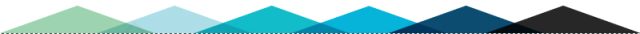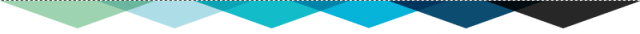25+48=（20+40+5+8=60+13=73

25+48=（25+40+8=65+8=73

25+48=（25+5+43=30+43=73

25+48=（25+45+3=70+3=73

…………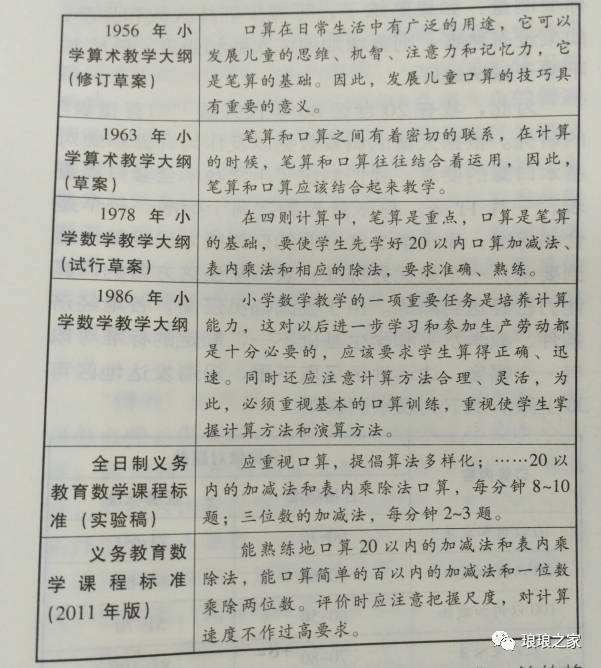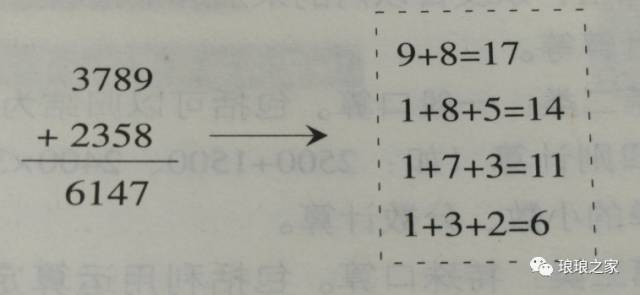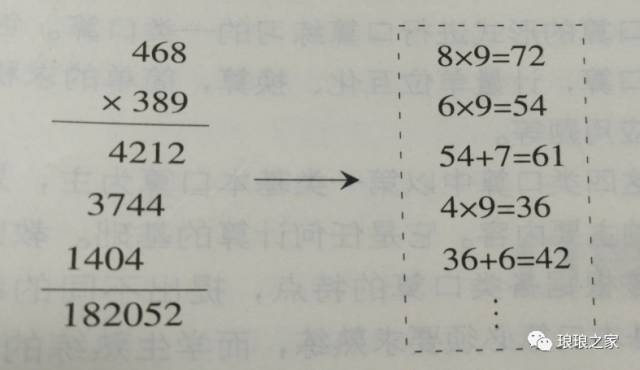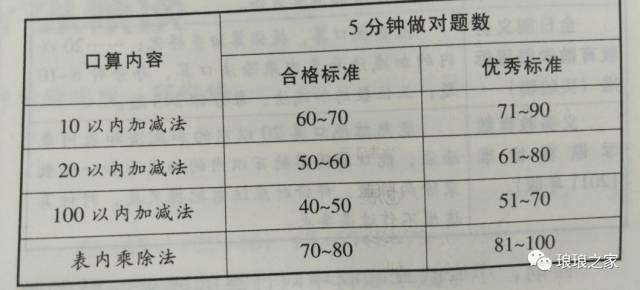（邱老师邮箱：13776884613@126.com）